# Introduction

voluModel contains several functions to generate formatted maps of rasters and points automatically. This was to aid us in cleanly visualizing large numbers of maps efficiently using ggplot2 (Wickam, 2016) and lattice (Sarkar, 2008) functionality. While there are quite a few features available in these functions, they were designed with fairly specific use cases in mind. You are welcome to gut the functions and rewrite them for your own custom needs.

The data I will be using for the demos in this tutorial will be loaded and analyzed first. The Luminous Hake occurrence points, which you can see in the data sampling tutorial, were downloaded via R (R Core Team, 2020) from GBIF (Chamberlain et al., 2021; Chamberlain and Boettiger, 2017) and OBIS (Provoost and Bosch, 2019) via occCite (Owens et al., 2021). The simple envelope model is based on two environmental datasets from the World Ocean Atlas (Garcia et al., 2019): temperature (Locarnini et al., 2018) and apparent oxygen utilization (AOU; Garcia et al., 2019). For more details on how to process environmental data, see the raster data tutorial. For the workflow used to generate the niche envelopes, refer to the introduction vignette.

library(voluModel) # Because of course
library(ggplot2) # For fancy plotting
library(viridisLite) # For high-contrast plotting palettes
library(dplyr) # To filter data
library(terra) # Now being transitioned in
library(sf) # Now being transitioned in

oxygenSmooth <- rast(system.file("extdata/oxygenSmooth.tif",
package='voluModel'))

package='voluModel'))

# Temperature
td <- tempdir()
unzip(system.file("extdata/woa18_decav_t00mn01_cropped.zip",
package = "voluModel"),
exdir = paste0(td, "/temperature"), junkpaths = T)
temperature <- vect(paste0(td, "/temperature/woa18_decav_t00mn01_cropped.shp"))

# Creating a SpatRaster vector
template <- centerPointRasterTemplate(temperature)
tempTerVal <- rasterize(x = temperature, y = template, field = names(temperature))

# Get names of depths
envtNames <- gsub("[d,M]", "", names(temperature))
envtNames[] <- "0"
names(tempTerVal) <- envtNames
temperature <- tempTerVal

# Oxygen processing
names(oxygenSmooth) <- names(temperature)

# Clean points ----
occurrences <- occs %>% dplyr::select(decimalLongitude, decimalLatitude, depth) %>%
distinct() %>% filter(dplyr::between(depth, 1, 2000))

# Gets the layer index for each occurrence by matching to depth
layerNames <- as.numeric(names(temperature))
occurrences$index <- unlist(lapply(occurrences$depth,
FUN = function(x) which.min(abs(layerNames - x))))
indices <- unique(occurrences$index) downsampledOccs <- data.frame() for(i in indices){ tempPoints <- occurrences[occurrences$index==i,]
tempPoints <- downsample(tempPoints, temperature[], verbose = FALSE)
tempPoints$depth <- rep(layerNames[[i]], times = nrow(tempPoints)) downsampledOccs <- rbind(downsampledOccs, tempPoints) } occsWdata <- downsampledOccs[,c("decimalLatitude", "decimalLongitude", "depth")] # Extract data ---- occsWdata$temperature <- xyzSample(occs = occsWdata, temperature)
## Using decimalLongitude, decimalLatitude, and depth
##  as x, y, and z coordinates, respectively.
occsWdata$AOU <- xyzSample(occs = occsWdata, oxygenSmooth) ## Using decimalLongitude, decimalLatitude, and depth ## as x, y, and z coordinates, respectively. occsWdata <- occsWdata[complete.cases(occsWdata),] # Land shapefile land <- rnaturalearth::ne_countries(scale = "small", returnclass = "sf") # Study region studyRegion <- marineBackground(occsWdata, buff = 1000000) ## Using decimalLongitude and decimalLatitude ## as x and y coordinates, respectively. # Get limits tempLims <- quantile(occsWdata$temperature,c(0, 1))
aouLims <- quantile(occsWdata$AOU,c(0, 1)) # Reclassify environmental bricks to presence/absence temperaturePresence <- classify(temperature, rcl = matrix(c(-Inf,tempLims[],0, tempLims[], tempLims[], 1, tempLims[], Inf, 0), ncol = 3, byrow = TRUE)) AOUpresence <- classify(oxygenSmooth, rcl = matrix(c(-Inf, aouLims[],0, aouLims[], aouLims[], 1, aouLims[], Inf, 0), ncol = 3, byrow = TRUE)) # Put it all together envelopeModel3D <- temperaturePresence * AOUpresence envelopeModel3D <- mask(crop(envelopeModel3D, studyRegion), mask = studyRegion) names(envelopeModel3D) <- names(temperature) rm(AOUpresence, downsampledOccs, occurrences, temperaturePresence, tempPoints, aouLims, envtNames, i, indices, layerNames, tempLims) # Plotting points There are two functions in voluModel for plotting occurrence points. The first, pointMap(), plots a single set of horizontal geographic points. The title generated is the species name the user supplies and a count of the number of points that have been plotted. pointMap(occs = occs, land = land, landCol = "black", spName = "Steindachneria argentea", ptSize = 2, ptCol = "orange")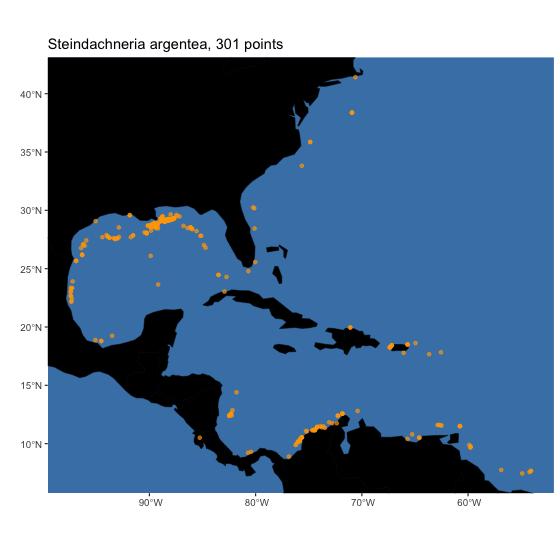The second, pointCompMap() plots a map that maps the extents of two sets of horizontal geographic points in different colors. This might be useful, for example, if you want to compare a raw dataset to a cleaned dataset. If any points overlap, they can be plotted as a third color. pointCompMap(occs1 = occs, occs1Col = "red", occs1Name = "Raw", occs2 = occsWdata, occs2Col = "orange", occs2Name = "Clean", spName = "Steindachneria argentea", agreeCol = "purple", land = land, landCol = "black", ptSize = 2, verbose = FALSE)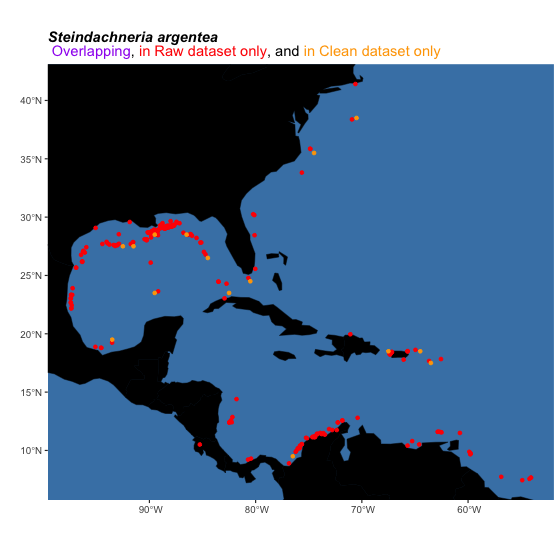# Plotting rasters If you have a horizontal raster you would like to map that contains continuous data, the function for you might be oneRasterPlot(). It is a wrapper around spplot() from sp (Pebesma and Bivand, 2005; Bivand et al. 2013) that implements the high-contrast, colorblind-friendly color palettes offered in viridis() from viridisLite (Garnier et al., 2021). The function optionally takes arguments to the spplot() and viridis() functions (so, for example, you can choose a palette other than the default). oneRasterPlot(rast = temperature[], land = land, title = "Sea Surface Temperature, WOA 2018", landCol = "black", n = 11, option = "mako", varName = "Temperature")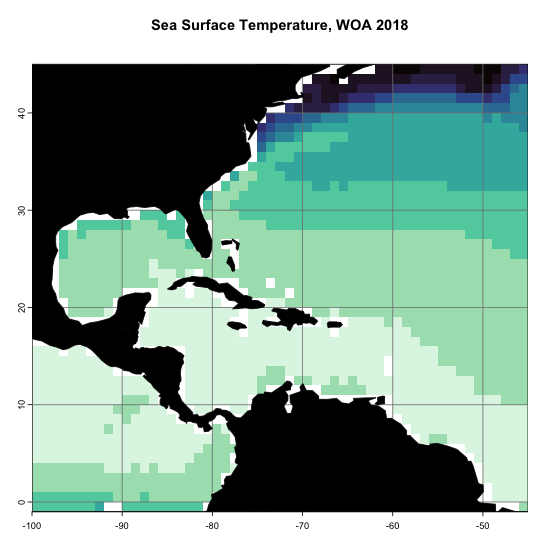Suppose you want to compare the extents of two presence-absence rasters–perhaps you are comparing two species distribution model method outputs, or comparing potential distributions at two different depths. We have a function for that, too. rasterComp(rast1 = envelopeModel3D[], rast1Name = "Surface", rast2 = envelopeModel3D[], rast2Name = "45m", land = land, landCol = "black", title = "Suitability of Habitat for Luminous Hake\nAt Two Different Depths")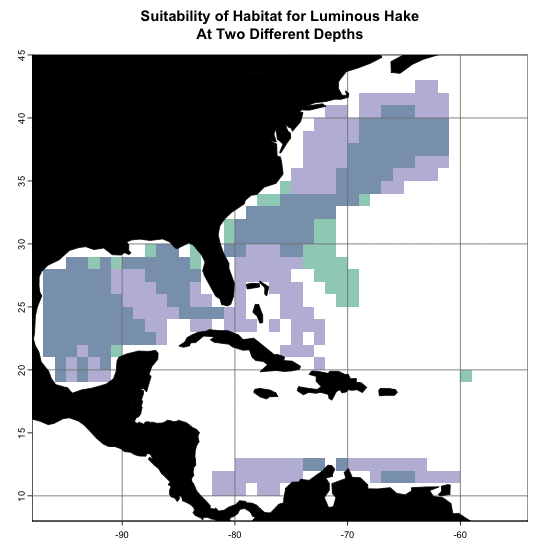Of course, 3D models such as the ones voluModel is designed to help you produce, are difficult to visualize. We have given it a try, though. plotLayers() plots a transparent layer of suitable habitat for each depth layer. The redder the color, the shallower the layer, the bluer, the deeper. The more saturated the color, the more layers with suitable habitat. Here, I am plotting suitability from 20 to 700 m, the depth range of occurrences used to train the envelope model. layerNames <- as.numeric(names(envelopeModel3D)) occsWdata$index <- unlist(lapply(occsWdata$depth, FUN = function(x) which.min(abs(layerNames - x)))) indices <- unique(occsWdata$index)

layerPlot <- plotLayers(envelopeModel3D[[min(indices):max(indices)]],
title = "Envelope Model of Luminous Hake,\n 20 to 700m",
land = land, landCol = "black")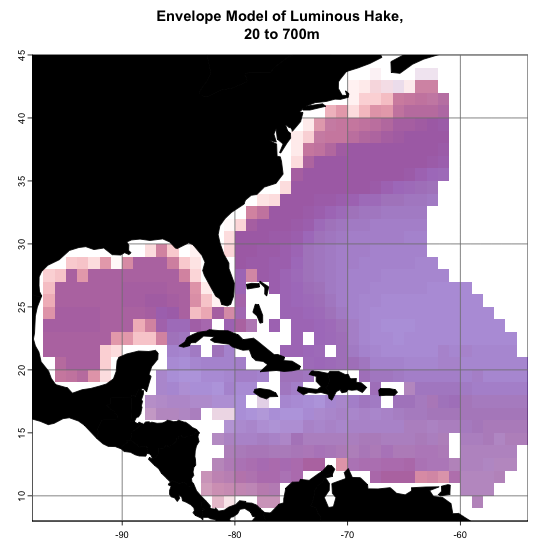# Tidying up

Last, we need to close the temporary directory we opened when we opened the data.

unlink(td, recursive = T)